GROUP THEORY TUTORIAL

# What's new in GTPack 1.1

GTPack version 1.1 was released on March 1st, 2020. Besides several bug fixes, GTPack 1.1 comes with a new sub package GTRepresentationTheorySG allowing for the calculation of representation matrices and character tables of space groups. It furthermore provides novel functionality for electronic structure calculations.

Handling and representation theory of space groups

GTPack 1.1 offers a few new modules to handle symmetry elements of space groups.

 GTSGgmat multiplies two space group elements GTSGOrderOfElement gives the order of a space group element
We will show the examples of this section for a monoclinic lattice.
 In:=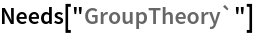In:=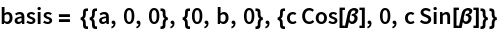Out=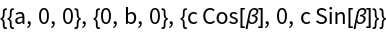In:=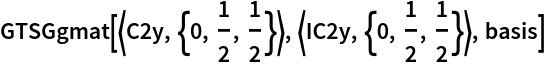Out=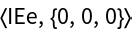In:=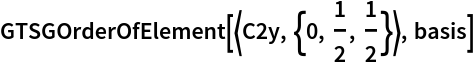Out=Additionally, GTPack 1.1 provides several new modules to analyze the group structure of space groups..

 GTSGClasses multiplies two space group elements GTSGGetInvSubGroup gives the order of a space group element GTSGLeftCosets gives the left cosets of the factor group G/T (G being a space group, T the group of pure lattice translations) with respect to a subgroup GTSGRightCosets gives the right cosets of the factor group G/T (G being a space group, T the group of pure lattice translations) with respect to a subgroup GTSGCosetRepresentative determines a coset representative for a left coset decomposition of the factor group G/T (G being a space group, T the group of pure lattice translations) into a normal subgroup of index 2 or 3
We choose the monoclinic space group P21/c (#14). We calculate the corresponding basis vectors of the reciprocal lattice using GTReciprocalBasis. Then we install the space groups using GTInstallGroup.
 In:=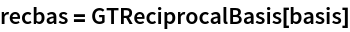Out=In:=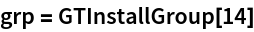Out=In:=Out=In:=Out=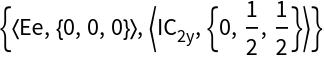We can use a coset representative to reconstruct a space group from an invariant subgroup.
 In:=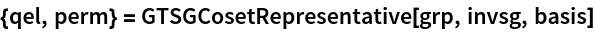Out=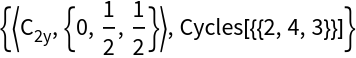In:=In:=Out=In:=Out=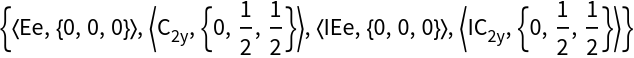In:=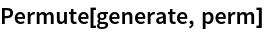Out=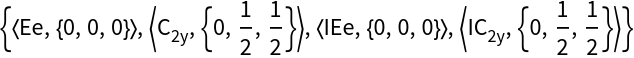Finally, we calculate the left and right cosets, which are identical for an invariant subgroup.
 In:=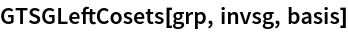Out=In:=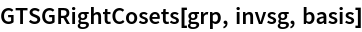Out=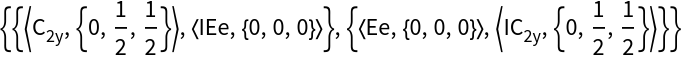The probably most important modules for space groups which were introduced in GTPack 1.1 concern the calculation of the irreducible representations and character table of space groups.

 GTSGCharacterTable gives the character table of a space group GTSGGetIreps gives the character table and representation matrices of a space group
 In:=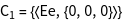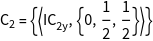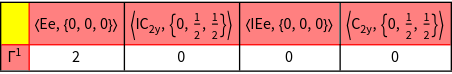Out=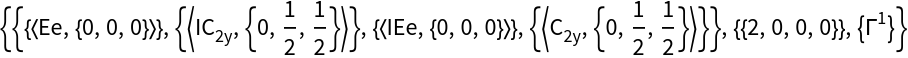In:=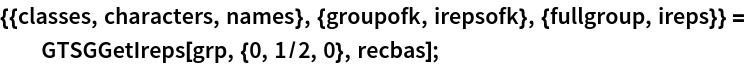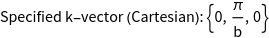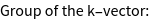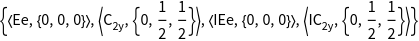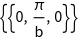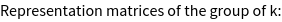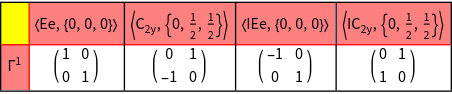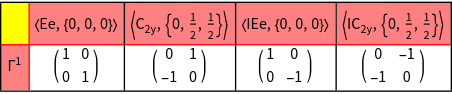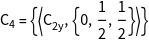In:=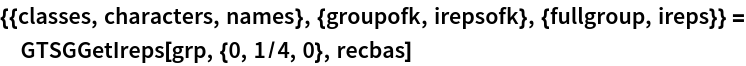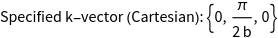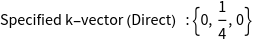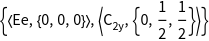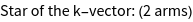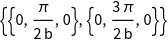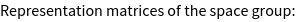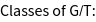Out=Exploring orbital characters in electronic structure calculations

GTPack 1.1 offers a new functionality to highlight the orbital character in electronic structure calculations.

 GTPartialDOS calculation of partial densities of states (TB Hamiltonians) GTFatBandsPlot plots orbital character of a band structure (TB Hamiltonians)
We show the new modules on the example of Cu. The corresponding data is loaded from an existing dataset. Furthermore, we generate a high-symmetry path for the fcc lattice using GTBZPath and the corresponding k-mesh using GTBZLines.
 In:=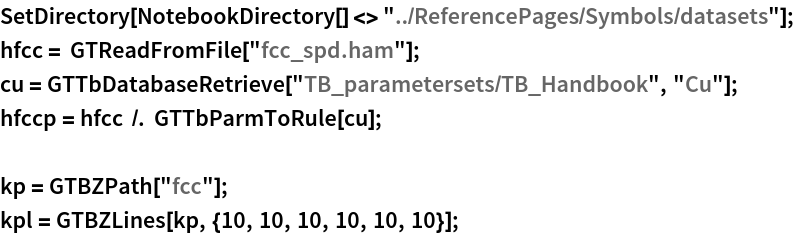To calculate fat bands the eigenvectors of the Hamiltonian are needed, which can be calculated using GTBands.
 In:=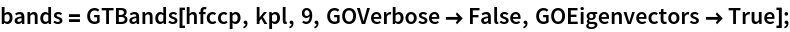In:=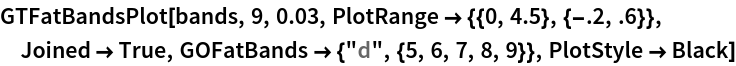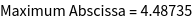Out=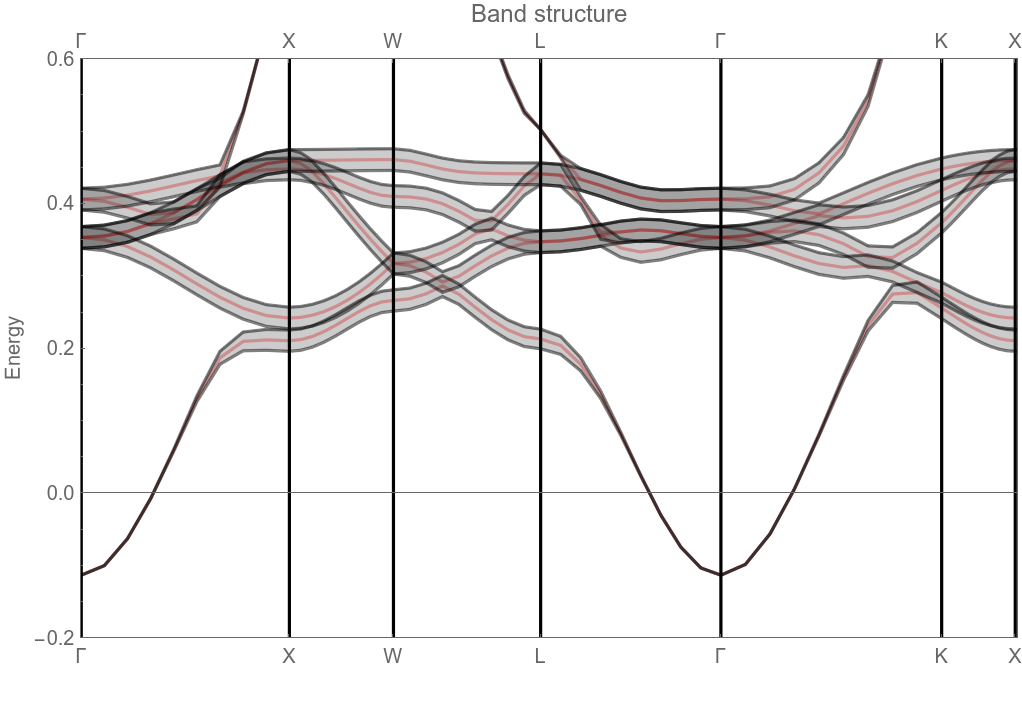Using the novel module GTPartialDOS we can also obtain the density of states for specified orbitals. For the partial DOS calculation, we provide a parameter list, where the last item describes which partial densities of states are calculated.
 In:=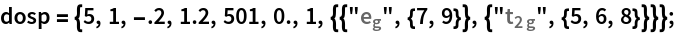In:=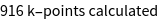Out=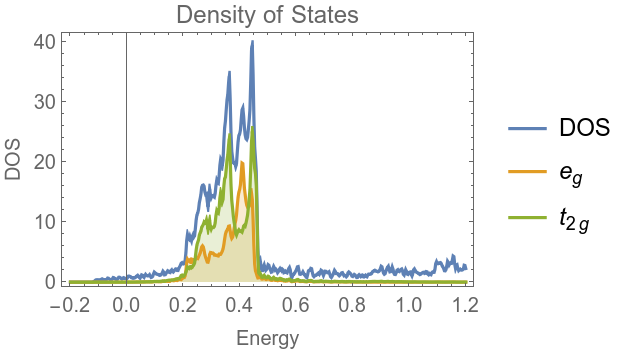Further modifications

Besides the new modules presented above, some existing modules where extended. For GTClusterManipulate, the new method "Impurities" was added, which allows to substitute a randomly chosen selection of one atomic species within a cluster by impurities. GTDensityOfStatesRS was extended to allow for eigenvalues of an electronic structure calculation as an input. This way, computationally demanding eigenvalue problems can be solved outside of Mathematica.